# Write complete ionic equation to show the reaction of aqueous Hg2(NO3)2 with aqueous sodium chloride to...

Write complete ionic equation to show the reaction of aqueous Hg2(NO3)2 with aqueous sodium chloride to form solid Hg2Cl2 and aqueous sodium nitrate.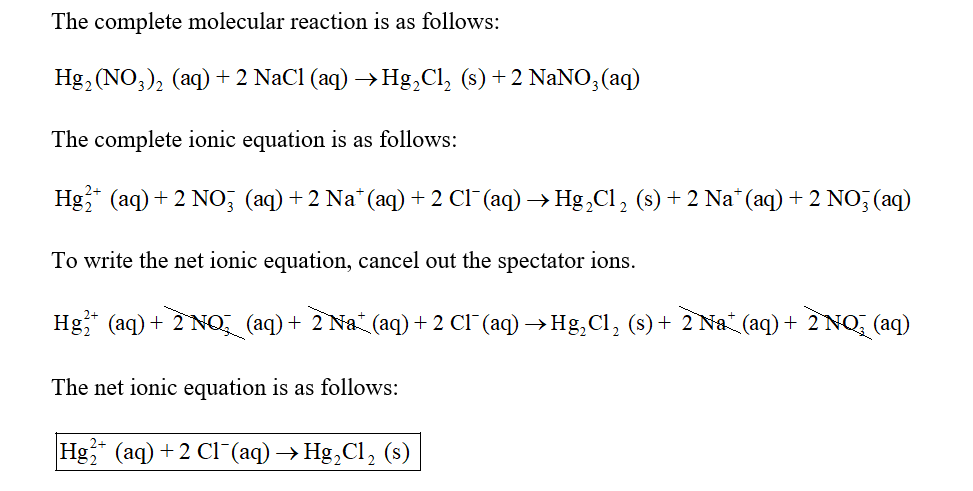#### Earn Coin

Coins can be redeemed for fabulous gifts.

Similar Homework Help Questions
• ### Complete and balance the molecular equation for the reaction of aqueous sodium carbonate and aqueous nickel Chloride In...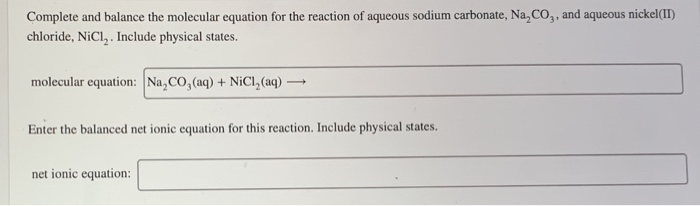Complete and balance the molecular equation for the reaction of aqueous sodium carbonate and aqueous nickel Chloride Include physical states Complete and balance the molecular equation for the reaction of aqueous sodium carbonate, Na, Co,, and aqueous nickel(II) chloride, NiCl. Include physical states. molecular equation: Na, CO, (aq) + NiCI, (aq) + Enter the balanced net ionic equation for this reaction. Include physical states. net ionic equation:

• ### write the molecular equation and the net ionic equation of each reaction. 20 7. barium chloride + sodium phosphate L...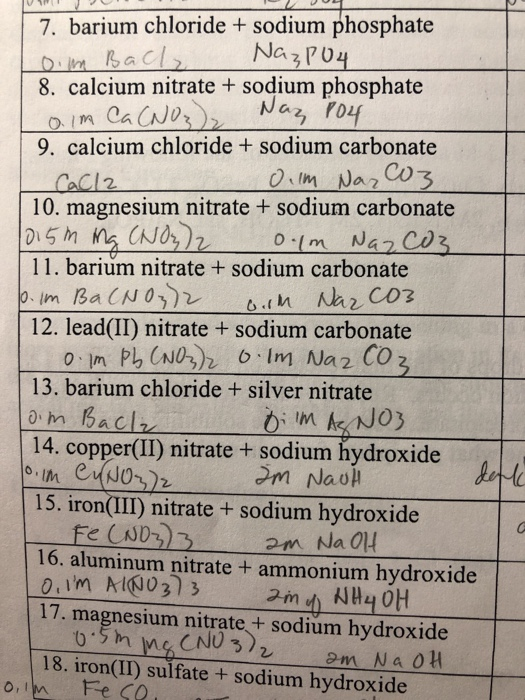write the molecular equation and the net ionic equation of each reaction. 20 7. barium chloride + sodium phosphate Loim Bach, Na₃PO4 8. calcium nitrate + sodium phosphate a im Ca (NO) Naz P04 9. calcium chloride + sodium carbonate CaCl2 Olm Nazo3 10. magnesium nitrate + sodium carbonate 1015m ng (NO3)2 0.1m Nazcos 11. barium nitrate + sodium carbonate 10. Im Ba(NO3)2 o.om Nazco3 12. lead(II) nitrate + sodium carbonate 0. Im Pb (NO3)2 0.Im NazC03 13. barium chloride...

• ### the balanced net ionic equation for the reaction of aqueous solutions of calcium chloride & sodium...

the balanced net ionic equation for the reaction of aqueous solutions of calcium chloride & sodium hydroxide is

• ### Give the  complete ionic equation for the reaction (if any) that occurs when aqueous solutions of sodium sulfide...

Give the  complete ionic equation for the reaction (if any) that occurs when aqueous solutions of sodium sulfide and iron(II) nitrate are mixed.

• ### > Complete and balance the molecular equation for the reaction of aqueous sodium sulfate, Na,SO,, and...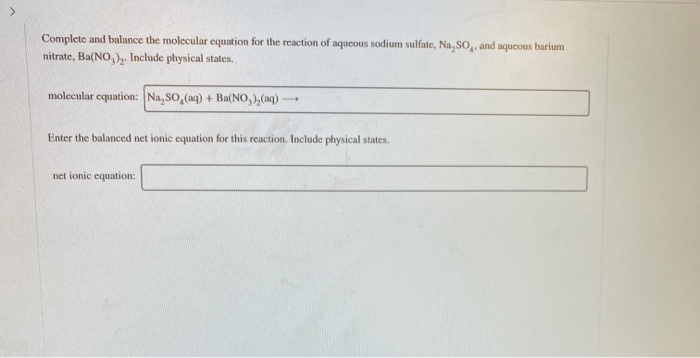> Complete and balance the molecular equation for the reaction of aqueous sodium sulfate, Na,SO,, and aqueous barium nitrate, Ba(NO,),. Include physical states. molecular cquation: Na,SO (aq) +Ba(NO,)(aq) Enter the balanced net ionic equation for this reaction. Include physical states. net ionic equation:

• ### ionic equation

Lead ions can be removed from solution by precipitation with sulfate ions. Suppose that a solution contains lead(II) nitrate.A. Write complete ionic equation to show the reaction of aqueous lead(II) nitrate with aqueous potassium sulfate to form solid lead(II) sulfate and aqueous potassiumnitrate."Express your answer as a chemical equation. Identify all of the phases in your answer"B. Write net ionic equation to show the reaction of aqueous lead(II) nitrate with aqueous potassium sulfate to form solid lead(II) sulfate and aqueous...

• ### i need the answers please 4. Write a balanced, ionic equation for the reaction that occurs when aqueous solutions of...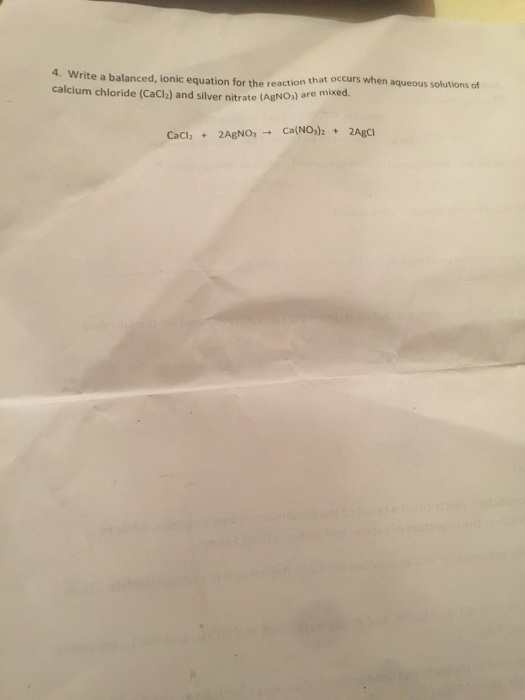i need the answers please 4. Write a balanced, ionic equation for the reaction that occurs when aqueous solutions of calcium chloride (CaCl2) and silver nitrate (AgNO) are mixed. CaCl2 + 2AgNO3 + Ca(NO3)2 + 2Agci

• ### Reaction 14: Aqueous iron(II) chloride + aqueous ammonium hydroxide Balanced Molecular Equation (from page 8): Complete...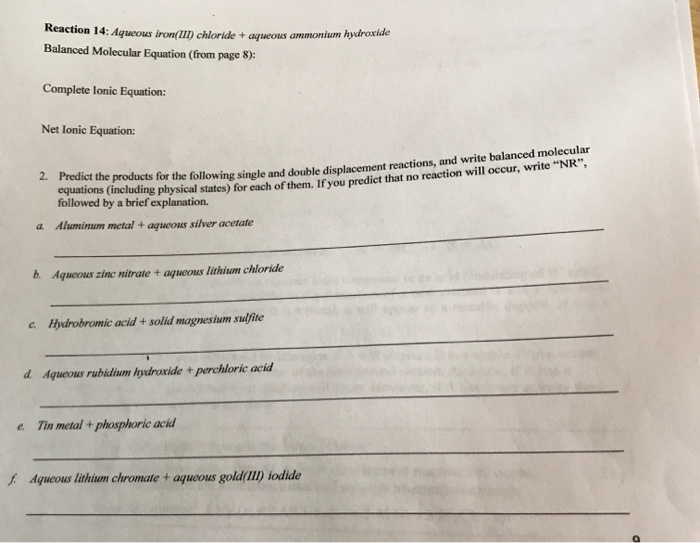Reaction 14: Aqueous iron(II) chloride + aqueous ammonium hydroxide Balanced Molecular Equation (from page 8): Complete lonic Equation: Net Ionic Equation: 2. Predict the products for the followine single and double displacement reactions, and wing single and double displacement reactions, and write balanced molecular squations including physical states) for each of them. If you predict that no reaction will occur, w na reaction will occur, write "NR", " followed by a brief explanation. a. Aluminum metal + aqueous silver acetate...

• ### Part A Write a balanced chemical equation for the reaction of aqueous sodium carbonate with aqueous copper(II) chlo...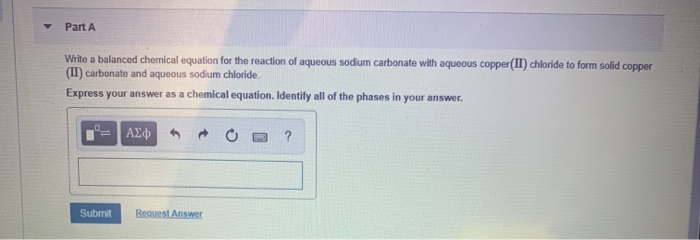Part A Write a balanced chemical equation for the reaction of aqueous sodium carbonate with aqueous copper(II) chloride to form solid copper (II) carbonate and aqueous sodium chloride. Express your answer as a chemical equation. Identify all of the phases in your answer. Submit Request Answer

• ### Write the molecular equation, ionic equation and net ionic equation for the following reaction: (Predict the spectator i...

Write the molecular equation, ionic equation and net ionic equation for the following reaction: (Predict the spectator ions) sodium chloride(aq) + silver nitrate(aq) → sodium nitrate(aq) + silver chloride(s)

Free Homework App